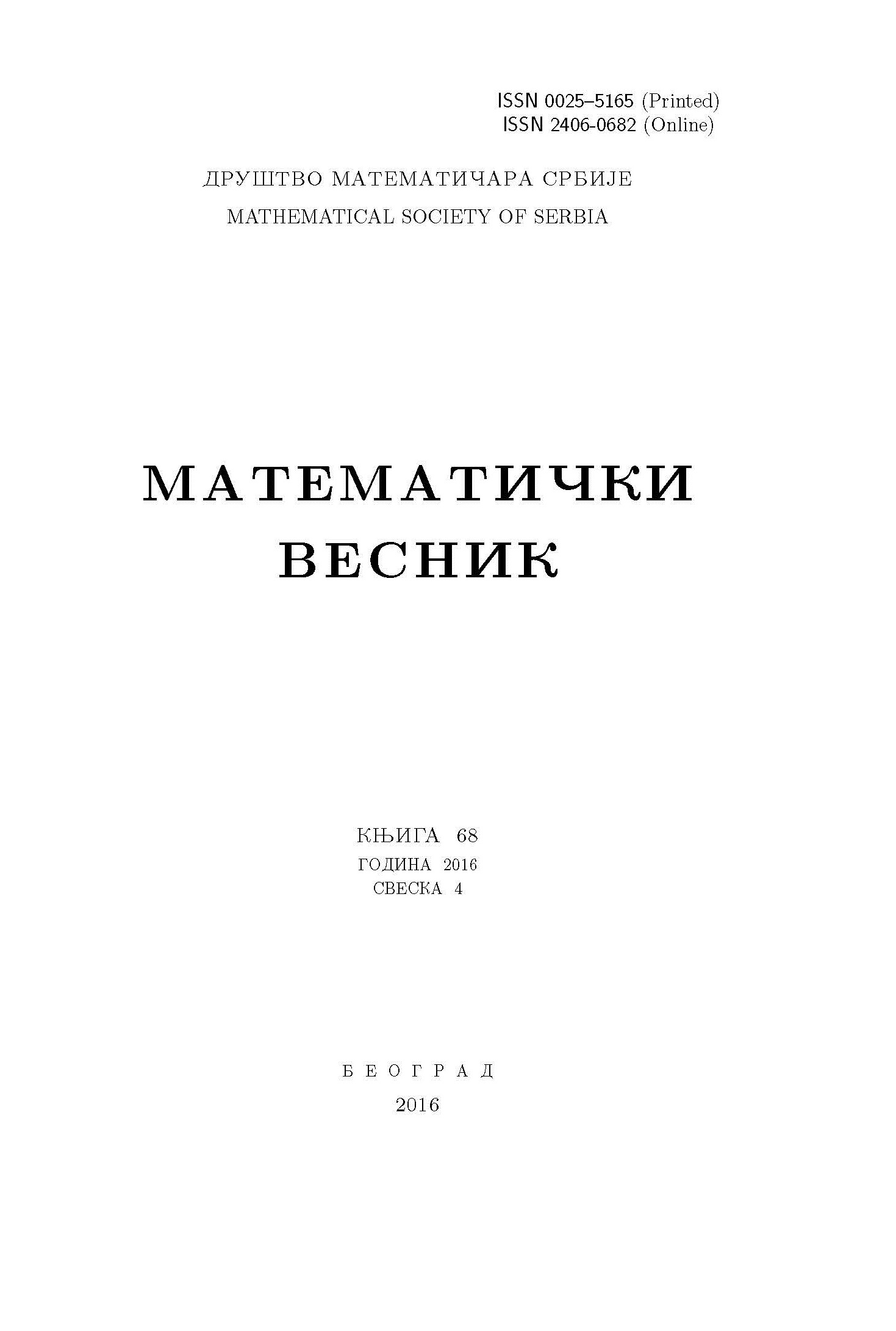﻿ Matematički Vesnik ﻿
 MATEMATIČKI VESNIK МАТЕМАТИЧКИ ВЕСНИКPROPERTIES OF ZERO-DIVISOR GRAPH OF THE RING $\mathbf{F}_{p^l} \times \mathbf{F}_{q^m} \times \mathbf{F}_{r^n}$ M. Nazim, N. Rehman AbstractIn this paper, we study some basic properties of the zero-divisor graph of ring $F_{p^l} \times F_{q^m} \times F_{r^n}$, where $F_{p^l}$, $F_{q^m}$ and $F_{r^n}$ are fields of order $p^l$, $q^m$ and $r^n$, respectively, $p, q$ and $r$ are primes (not necessarily distinct) and $l, m, n \geq 1$ are positive numbers. Also, we discuss some topological indices of the graph $\Gamma(F_{p^l} \times F_{q^m} \times F_{r^n})$.Keywords: Zero-divisor graph; direct product of rings; graph parameters.. MSC: 05C10, 05C12, 05C25 Pages:  1$-$10

﻿